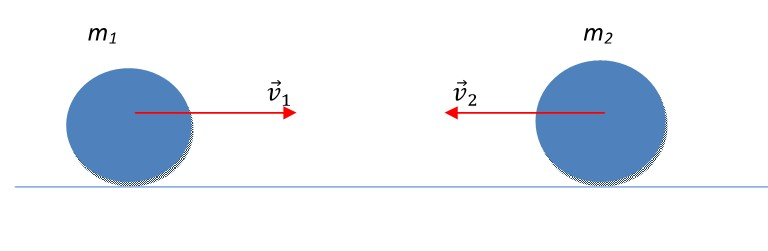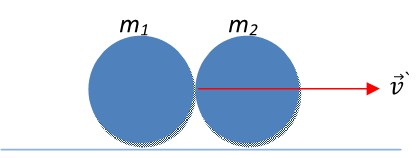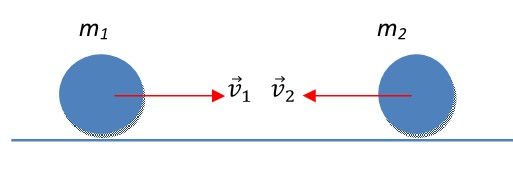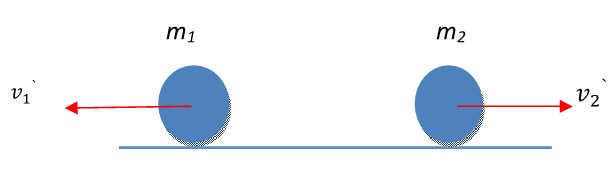﻿ All the formulas related to Conservation Laws

# List of formulas related to Conservation law

## Momentum of Force

$$\vec{p} = m\vec{v}$$

### Law of conservation of momentum

V1, V2: initial velocities of objects 1 and 2; V1, V2: final velocities of objects 1 and 2

$$\vec{p} = \vec{p'} => m_1\vec{v_1} + m_2\vec{v_2} = m_1\vec{v'_1} + m_2\vec{v'_2}$$

### Elastic Collision

V: Velocity of the two body system(m1+m2) after impact$${v'} = \frac{m_1 {v_1} + m_2 {v_2}}{m_1 + m_2}$$

### One Dimensional Elastic Collision

v1, v2`: velocities of m1 and m2 after impact$${v'_1} = \frac{(m_1 - m_2){v_1} + 2 m_2 {v_2}}{m_1 + m_2}$$ $${v'_2} = \frac{(m_2 - m_1){v_2} + 2 m_1 {v_1}}{m_1 + m_2}$$